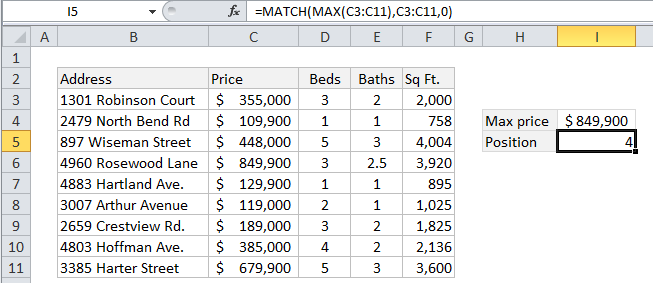## Excel Office

Excel How Tos, Tutorials, Tips & Tricks, Shortcuts

# Position of max value in list in Excel

This tutorial shows how to  calculate Position of max value in list in Excel  using the example below;

## Formula

`=MATCH(MAX(range),range,0)`## Explanation

To get the position of the maximum value in a range (i.e. a list, table, or row), you can use the MAX function together with the MATCH function.

In the example shown, the formula in I5 is:

`=MATCH(MAX(C3:C11),C3:C11,0)`

Which returns the number 4, representing the position in this list of the the most expensive property.

Also See:   Join tables with INDEX and MATCH in Excel

### How this formula works

The MAX function first extracts the maximum value from the range C3:C11.

In this case, that value is 849900.

This number is then supplied to the MATCH function as the lookup value. The lookup_array is the same range C3:C11, and the match_type is set to “exact” with 0.

Also See:   Get column index in Excel Table

With those arguments, MATCH locates finds the maximum value inside the range and returns the relative position of the value in that range.

In this case, the position corresponds to a relative row number, but in a horizontal range, the position would correspond to a relative column number.

Note: in case of duplicates (i.e. two or more max values that are the same) this formula will return the position of the first match, the default behavior of the MATCH function.

Also See:   Check if range contains a value not in another range in Excel